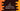# 3 different C programs to find all prime numbers in a range## C program to find all prime numbers in a range:

In this post, we will write a program to find all prime numbers in a given range or it will find all the prime numbers between 1 and N, where N will be a user-given number. The program will ask the user to enter the value of N and it will print all numbers between 1 to N.

With this program, you will learn how to check if a number is prime or not, and how to use a loop to find all prime numbers in a given range.

### Prime number:

A number is called a prime number if it is divisible by 1 and the number itself. For example, 7 is a prime number but 8 is not. Note that 1 is not a prime number. The first prime number is 2. It is also the only even prime number because all other even numbers are divisible by 2.

### Algorithm to check if a number is prime or not:

To check if a number is prime or not, we have to use a loop. The loop will check for all numbers starting from 2. If it finds any value that can divide the number, it will not be a prime number. Else, it will be a prime number.

We can iterate from 2 to number - 1. We can also iterate from 2 to number/2 to reduce the number of iteration. Because no number greater than number/2 can divide the number. Similarly, we can further optimize it to iterate from 2 to square root of number. Let’s write down programs for all these ways.

### Method 1: C program to find all prime numbers between 1 to N:

In this method, we will use a loop to iterate from 2 to number - 1 to check if a number is prime or not. Below is the complete program:

``````#include <stdio.h>

int isPrime(int no)
{
if (no == 1)
{
return 0;
}
for (int i = 2; i < no; i++)
{
if (no % i == 0)
{
return 0;
}
}
return 1;
}

int main()
{
int N;

printf("Enter the value of N: ");
scanf("%d", &N);

printf("Prime numbers between 1 to %d: ", N);

for (int i = 2; i <= N; i++)
{
if (isPrime(i))
{
printf("%d ", i);
}
}
}``````

Here,

• N is a variable to hold the upper limit of the range. It asks the user to enter the value of N, and stores the value in N.
• The for loop prints the prime numbers between 1 to N. Since 1 is not a prime number, the loop starts from 2.
• The isPrime method checks if the current value of i is prime or not. If yes, it prints the value of i.
• The isPrime method takes one number as its parameter and checks if that number is prime or not. It returns 0 if it is not a prime number. Else, it returns 1.
• If the number is 1, it returns 0.
• It runs one loop from i = 2 to i = number - 1. On each iteration, it checks if the number is divisible by i or not. If it is divisible, it returns 0 i.e. it is not a prime number. Once the loop ends, it returns 1, i.e. it is a prime number.

If you run this program, it will print output as like below:

``````Enter the value of N: 100
Prime numbers between 1 to 100: 2 3 5 7 11 13 17 19 23 29 31 37 41 43 47 53 59 61 67 71 73 79 83 89 97

Enter the value of N: 10
Prime numbers between 1 to 10: 2 3 5 7``````

### Method 2: C program to find all prime numbers between 1 to N by iterating to number/2:

We can iterate from 2 to number/2 to check if a number is prime or not. Let’s change the isPrime method of the above example to run the loop from 2 to number/2:

``````#include <stdio.h>

int isPrime(int no)
{
if (no == 1)
{
return 0;
}
for (int i = 2; i <= no/2; i++)
{
if (no % i == 0)
{
return 0;
}
}
return 1;
}

int main()
{
int N;

printf("Enter the value of N: ");
scanf("%d", &N);

printf("Prime numbers between 1 to %d: ", N);

for (int i = 2; i <= N; i++)
{
if (isPrime(i))
{
printf("%d ", i);
}
}
}``````

If you run this program, it will give a similar output.

### Method 3: C program to find all prime numbers between 1 to N by iterating to square root of number:

Let’s reduce the number of iteration further. Instead of iterating to number/2, we can also iterate up to square root of the number to check if a number is prime or not. Below is the complete program:

``````#include <stdio.h>
#include <math.h>

int isPrime(int no)
{
if (no == 1)
{
return 0;
}
for (int i = 2; i <= sqrt(no); i++)
{
if (no % i == 0)
{
return 0;
}
}
return 1;
}

int main()
{
int N;

printf("Enter the value of N: ");
scanf("%d", &N);

printf("Prime numbers between 1 to %d: ", N);

for (int i = 2; i <= N; i++)
{
if (isPrime(i))
{
printf("%d ", i);
}
}
}``````

This program uses the sqrt method to find the square root of a number. This method is defined in the math.h header file. It will print similar result.